# Overview of different Optimizers for neural networks

Source: Deep Learning on Medium

In this post we will start understand the objective of machine Learning algorithms. How Gradient Descent helps achieve goal of the the machine learning . Understand the role of optimizers in Neural networks. Explore different optimizers like Momentum, Nesterov, Adagrad, Adadelta, RMSProp, Adam and Nadam.

### Objective of Machine Learning algorithm

Goal of machine learning and deep learning is to reduce the difference between the predicted output and the actual output. This is also called as Cost function(C) or Loss function. Cost functions are a convex functions.

As our goal is to minimize the cost function by finding the optimized value for weights. We also need to ensure that the algorithm generalize well. This will help make better prediction for the data that was not seen before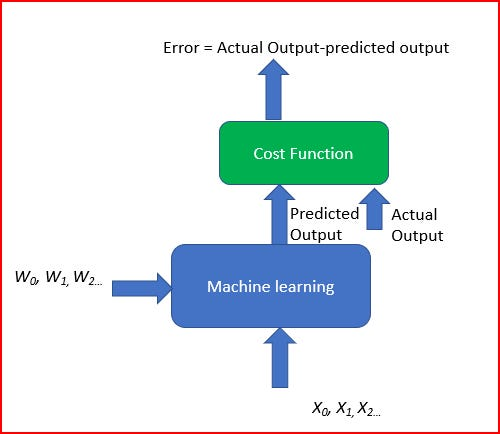Gradient based learning

To achieve this we run multiple iterations with different weights. This helps to find the minimum cost. This is Gradient descent.

### Gradient Descent

Gradient descent is an iterative machine learning optimization algorithm to reduce the cost function. This will help models to make accurate predictions.

Gradient indicates the direction of increase. As we want to find the minimum point in the valley we need to go in opposite direction of the gradient.We update parameters in the negative gradient direction to minimize the loss.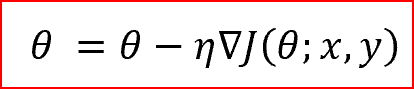θ is the weight parameter, η is the learning rate and ∇J(θ;x,y) is the gradient of weight parameter θ

### Types of Gradient Descent

Different types of Gradient descents are

• Batch Gradient Descent or Vanilla Gradient Descent
• Stochastic Gradient Descent
• Mini batch Gradient Descent

Read details of the different types of Gradient Descent here

### Role of an optimizer

Optimizers update the weight parameters to minimize the loss function. Loss function acts as guides to the terrain telling optimizer if it is moving in the right direction to reach the bottom of the valley, the global minimum.

### Types of Optimizers

#### Momentum

Momentum is like a ball rolling down hill. The ball will gain momentum as it rolls down the hill.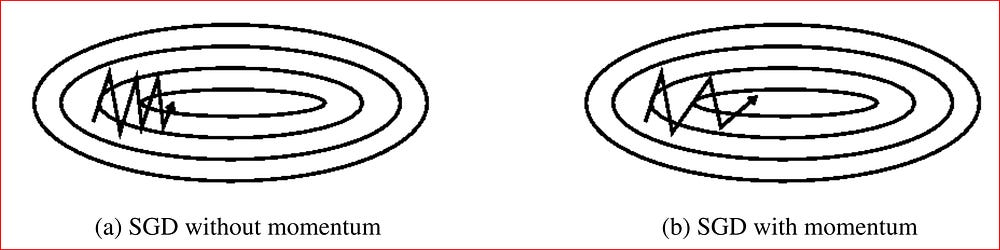Source: Genevieve B. Orr

Momentum helps accelerate Gradient Descent(GD) when we have surfaces that curves more steeply in one direction than in another direction. It also dampens the oscillation as shown above

For updating the weights it takes the gradient of the current step as well as the gradient of the previous time steps. This helps us move faster towards convergence.

Convergence happens faster when we apply momentum optimizer to surfaces with curves.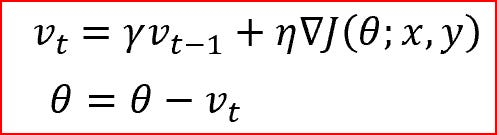Momentum Gradient descent takes gradient of previous time steps into consideration

#### Nesterov accelerated gradient(NAG)

Nesterov acceleration optimization is like a ball rolling down the hill but knows exactly when to slow down before the gradient of the hill increases again.

We calculate the gradient not with respect to the current step but with respect to the future step. We evaluate the gradient of the looked ahead and based on the importance then update the weights.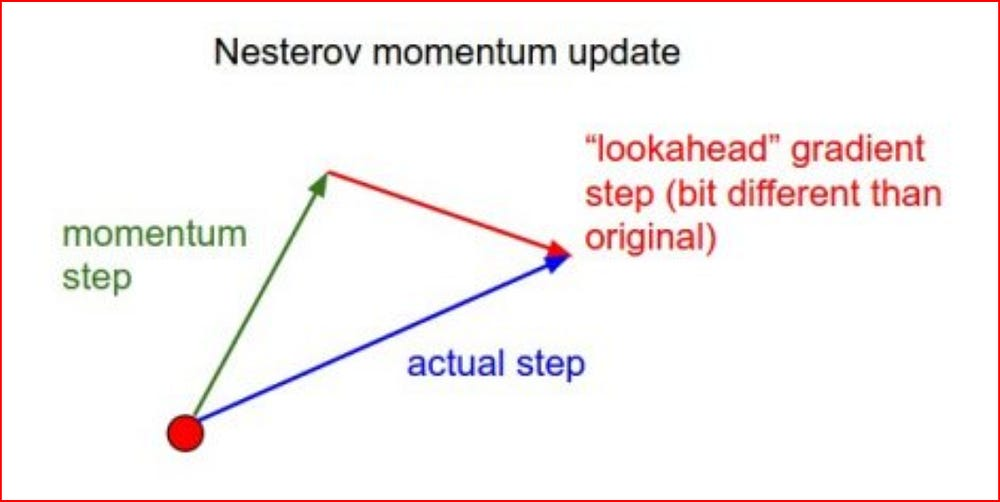source:http://cs231n.github.io/neural-networks-3/

NAG is like you are going down the hill where we can look ahead in the future. This way we can optimize our descent faster. Works slightly better than standard Momentum.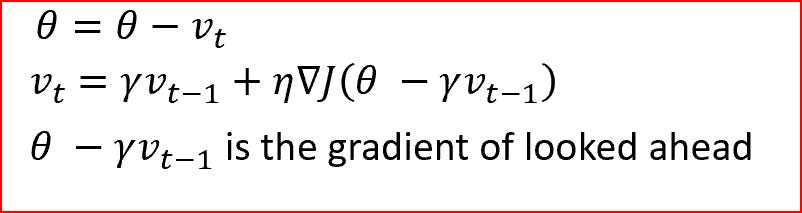Nesterov Accelerated Gradient

#### Adagrad — Adaptive Gradient Algorithm

We need to tune the learning rate in Momentum and NAG which is an expensive process.

Adagrad is an adaptive learning rate method. In Adagrad we adapt the learning rate to the parameters. We perform larger updates for infrequent parameters and smaller updates for frequent parameters.

It is well suited when we are have sparse data as in large scale neural networks. GloVe word embedding uses adagrad where infrequent words required greater update and frequent words require smaller updates.

For SGD, Momentum and NAG we update for all parameters θ at once. We also use the same learning rate η. In Adagrad we use different learning rate for every parameter θ for every time step t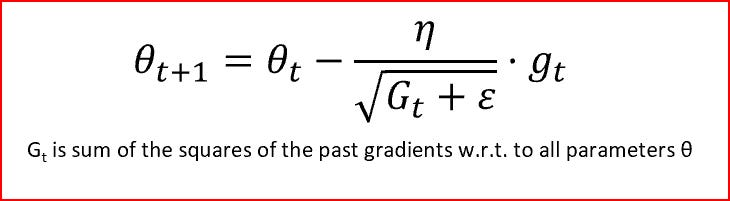Adagrad

Adagrad eliminates the need to manually tune the learning rate.

In the denominator we accumulate the sum of square of the past gradients. Each term is a positive term so it keeps on growing making the learning rate η infinitesimally small to the point that algorithm is no longer able learning. Adadelta, RMSProp and adam tries to resolve Adagrad’s radically diminishing learning rates.

#### Adadelta

• Adadelta is an extension of Adagrad and it also tries to reduce Adagrad’s aggressive, monotonically reducing learning rate
• It does this by restricting the window of past accumulated gradient to some fixed size of w. Running average at time t then depends on the previous average and the current gradient
• In Adadelta we do not need to set the default learning rate as we take the ratio of the running average of the previous time steps to the current gradient

#### RMSProp

• RMSProp is Root Mean Square Propagation. It was devised by Geoffrey Hinton.
• RMSProp tries to resolve Adagrad’s radically diminishing learning rates by using a moving average of squared gradient. It utilizes the magnitude of the recent gradient descents to normalize the gradient.
• In RMSProp learning rate gets adjusted automatically and it chooses a different learning rate for each parameter.
• RMSProp divides the learning rate by the average of the exponential decay of squared gradients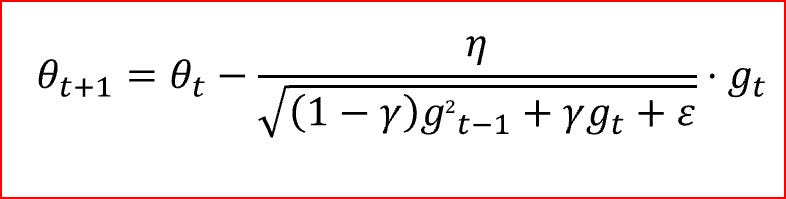γ is the decay term that takes value from 0 to 1. gt is moving average of squared gradients

#### Adam — Adaptive Moment Estimation

• Another method that calculates the individual adaptive learning rate for each parameter from estimates of first and second moments of the gradients.
• It also reduces the radically diminishing learning rates of Adagrad
• Adam can be viewed as a combination of Adagrad, which works well on sparse gradients and RMSprop which works well in on-line and non stationary settings.
• Adam implements the exponential moving average of the gradients to scale the learning rate instead of simple average as in Adagrad. It keeps an exponentially decaying average of past gradients
• Adam is computational efficient and has very little memory requirement
• Adam optimizer is one of the most popular gradient descent optimization algorithms

Adam algorithm first updates the exponential moving averages of the gradient(mt) and the squared gradient(vt) which is the estimates of the first and second moment.

Hyper-parameters β1, β2 ∈ [0, 1) control the exponential decay rates of these moving averages as shown below

Moving averages are initialized as 0 leading to moment estimates that are biased around 0 especially during the initial timesteps. This initialization bias can be easily counteracted resulting in bias corrected estimates

Finally we update the parameter as shown below

#### Nadam- Nesterov-accelerated Adaptive Moment Estimation

• Nadam combines NAG and Adam
• Nadam is employed for noisy gradients or for gradients with high curvatures
• Learning process is accelerated by summing up the exponential decay of the moving averages for the previous and current gradient

In the diagram below we see can see how different optimizer will converge to the minimum. Adagrad, Adadelta, and RMSprop headed off immediately in the right direction and converge. Momentum and NAG were led off-track, evoking the image of a ball rolling down the hill. NAG corrected itself quickly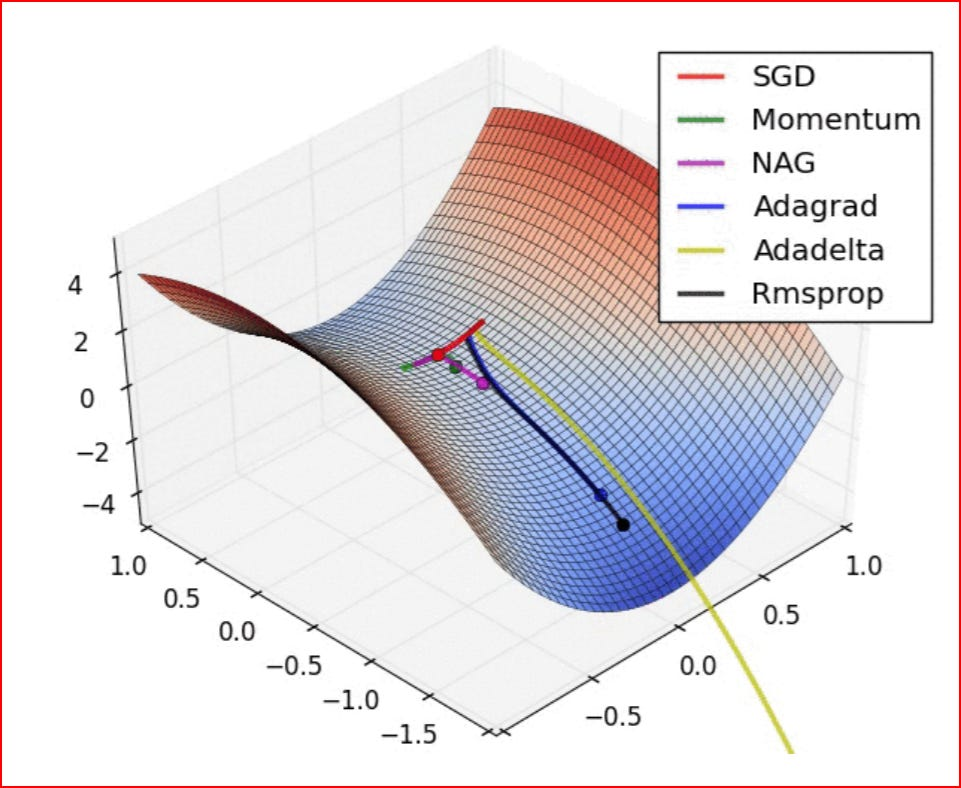Source Source and full animations: Alec Radford

#### References:

Adam: A Method for Stochastic Optimization by Diederik P. Kingma, Jimmy Ba

http://cs231n.github.io/neural-networks-3/

https://arxiv.org/pdf/1609.04747.pdf

http://yann.lecun.com/exdb/publis/pdf/lecun-98b.pdf